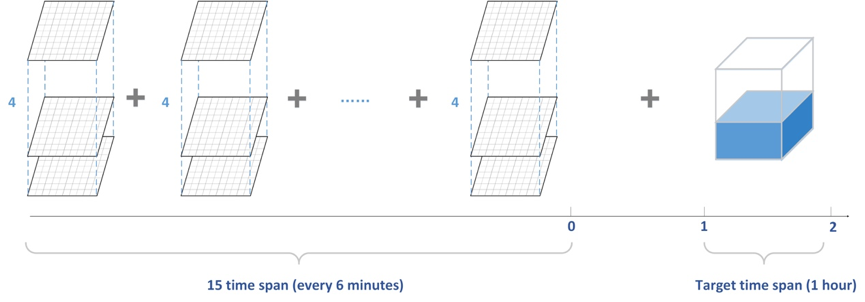# CIKM_AnalytiCup_2017: Combining the Traditional Methods and Deep Learning Approach for Precipitation Nowcasting

This repo discribes the solution of Team 怀北村明远湖. CIKM AnalytiCup 2017 is an open competition that is sponsored by Shenzhen Meteorological Bureau, Alibaba Group and CIKM2017. Our team got the third place in the first phrase. And in the second phrase we got the fourth place.

# Introduction

Short-term precipitation forecasting such as rainfall prediction is a task to predict a short-term rainfall amount based on current observations. In this challenge, sponsors provide a set of radar maps at different time spans where each radar map covers radar reflectivity of a target site and its surrounding areas. Radar maps are measured at different time spans, i.e., 15 time spans with an interval of 6 minutes, and different heights, i.e., 4 heights, from 0.5km to 3.5km with an interval of 1km; Each radar map covers an area of 101km*101km around the site. The area is marked as 101*101 grids, and the target site is located at the centre, i.e. (50, 50).

Our task here is to predict the total rainfall amount on the ground between future 1-hour and 2-hour for each target site.In this challenge, we combine Random Forestry, XGBoost and Bidirectional Gated Recurrent Units (GRUs) into an ensemble model to tackle this problem and achieve satisfying result.# Data Process

### Percentile Method

A statistical method was applied to reduce the dimension of radar data. For a single radar map, we pick the 25th, 50th, 75th, 100th percentile of reflectivity values in various scales of neighborhood around the target site from center to the whole map.### “Wind” Methond

We first handle the original data (15*4*101*101) into a small size of data (15*4*10*10). Then shrink the data into 15*4*6*6 features through judging the wind direction. The entire preprocess learns from the idea of CNN, especially the convolutional calculation and max polling.We take the fourth layer of data to determine the wind direction. Then, in order to calculating the resulting wind direction, we carry out two ways of choosing representative data. The first one uses the maximum value in each 10*10 frame as the representation. The second one takes the average of the largest five data instead. After selecting the representative data, we determine the wind direction by calculating the deviation between the initial position and the following frames, voting the moving direction, finally get the maximum votes as the resulting wind direction based on the given thresholds.# Model

Random Forestry, XGBoost and Bidirectional GRUs are utilized for model ensemble.

• Python 3.6
• Keras
• XGBoost
• sklearn